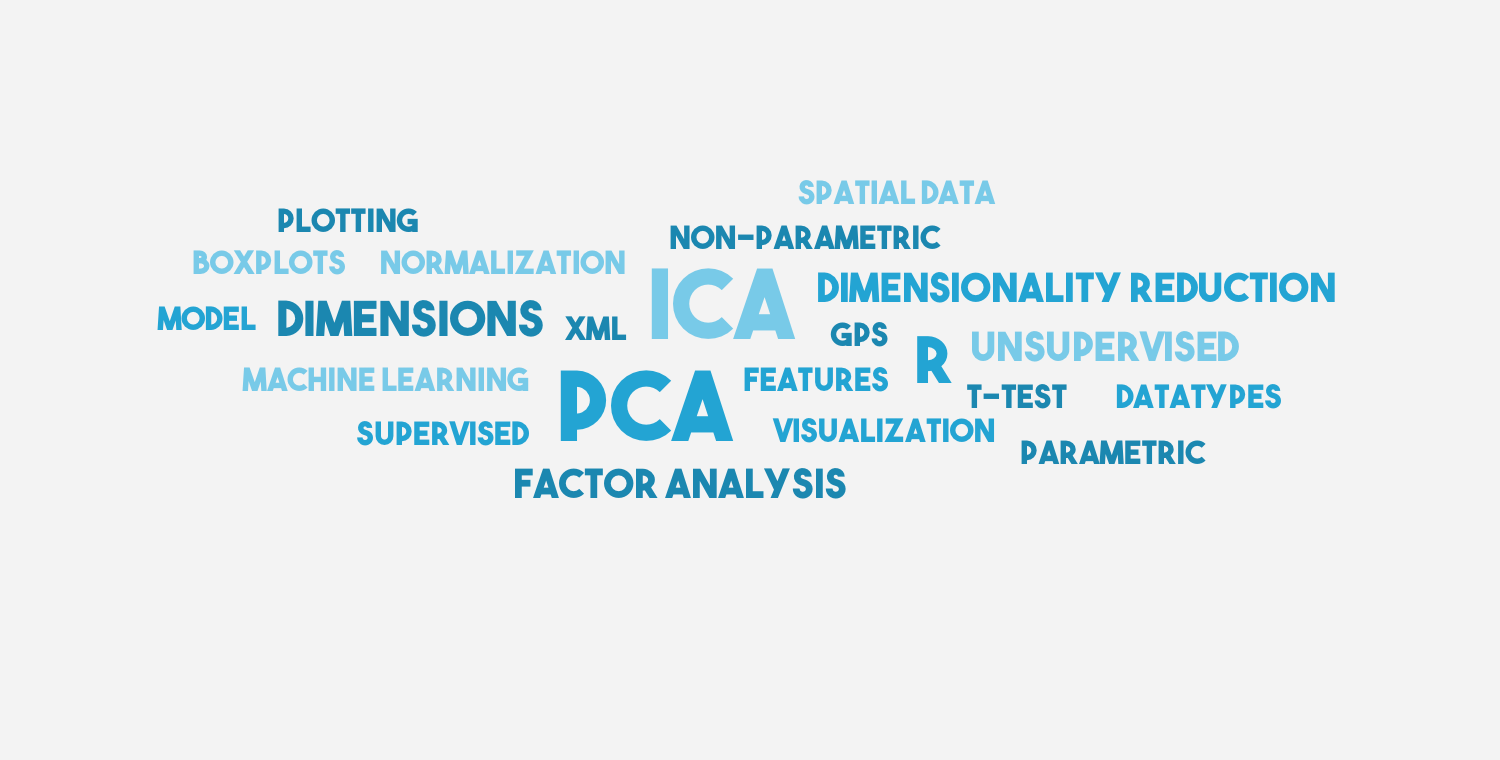# [Dimensionality Reduction #1] Understanding PCA and ICA using R

This time I am going to show you how to perform PCA and ICA. In the next one or two posts I will show you Factor Analysis and some scaling and projection methods.

The idea for this mini-series was inspired by a Machine Learning (Unsupervised) lecture I had at university.

I will perform all this methods on the same data sets and compare the results. I might use some additional self generated datasets to show properties of each method. The focus of these posts should not be on the R code (because it is very simple and the methods do not have many parameters) but on the interpretation, which is most important and can best be shown with visualisation.

The source code for this post can be downloaded here.

### Motivation

Some data has many dimensions / features, but for classification often a small subset of dimensions would be sufficient, therefore different dimensionality reduction methods can be applied to find a meaningful subset.

### Data

For showing these unsupervised techniques I use the wine dataset, consisting of 3 classes (different cultivars) and 13 features (quantities of components).

winedata=read.csv("wine.data.txt",sep=",", header=F)
wine_s<-scale(winedata[,2:14])


### Principal Component Analysis (PCA)

PCA is a method to find variance in the data. It transforms the coordinate system such that the data has the highest variance along the first component, the second highest along the second component, ...

Hint: You get as many principal components as your data has dimensions, but most of the variance is usually captured in the first few components, hence dimensionality reduction.
library(scatterplot3d)
pc<-princomp(wine_s)
summary(pc)

pairs(pc$scores[,1:5], col=rainbow(3)[winedata[,1]], asp=1) scatterplot3d(pc$scores[,c(1,2,4)], color=rainbow(3)[winedata[,1]])


The output of summary is the following (showing only the first 4 components, there are 13 altogether):

Importance of components:

 Comp.1 Comp.2 Comp.3 Comp.4 Standard deviation 2.1631951 1.5757366 1.1991447 0.9559347 Proportion of Variance 0.3619885 0.1920749 0.1112363 0.0706903 Cumulative Proportion 0.3619885 0.5540634 0.6652997 0.7359900

This tells us that the first component explains 36.1 % of the variance, component 2 explains 19 % of the variance and so on...

The first graph shows the pairwise plots of the first 5 principal components (PCs). Each plot can be seen as a transformation in a 2-dimensional space where the 2 components are the new coordinate system.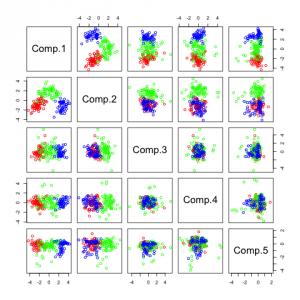We see that projection along the first PC separates the three classes pretty good, the best result seems to be a combination of 1st and 4th component.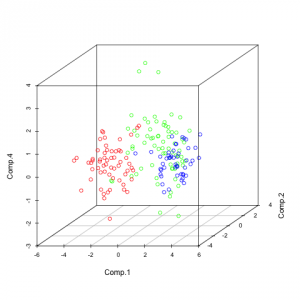A 3D representation of PCs 1, 2 and 4, which seem to be the most useful for me with regards to separating the 3 classes.
Note: Although PCA is very useful in finding the components with the highest variation, it does not always mean that the component are useful for separation in that order.

#### Representation of principal components on a random dataset

For better understanding I plotted the PCs I received (but on a different dataset). It is a good dataset to show how PCA works because you can clearly see that the data varies most along the first principal component. Since it is a 2-dimensional dataset, the second PC is simply the orthogonal vector to the first PC.

Hint: If you want to create such a plot, keep the aspect ratio at 1, (asp=1), than it can be seen that the components are orthogonal.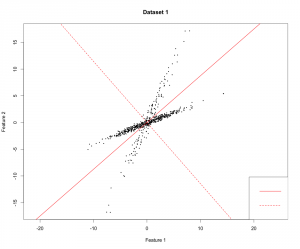First component (solid) and second component (dashed) represented by the red lines.

### Independent Component Analysis (ICA)

The purpose of ICA is to find independent components in the data. In contrast to PCA you do not automatically get the same amount of components as you have dimensions. Independent components also do not have to be orthogonal and they are not ranked (there is no "most independent component").

library(fastICA)
ica<-fastICA(wine_s, n.comp=5)
ica8<-fastICA(wine_s, n.comp=8)

pairs(ica$S, col=rainbow(3)[winedata[,1]]) pairs(ica8$S, col=rainbow(3)[winedata[,1]])

plot(ica$S[,1], ica$S[,1], col=rainbow(3)[winedata[,1]], xlab="Comp 1", ylab="Comp 1")


You see that I used a parameter called n.comp which tells the method how many independent components I want. The first two plots show this:

Not many of these components seem useful, in the first figure the 2 most useful components with regards to separation seem to be IC1 and IC5. In the second figure it seems to be IC1 and IC8. If you look closely you can see that these two components are the same in the 2 figures. IC1 (first fig.) and IC8 (second fig.) seems to be able to separate the data on its own.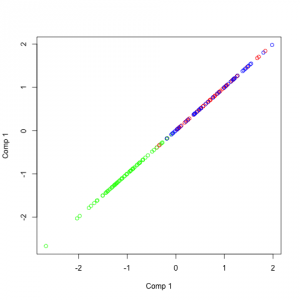IC1 / IC8 plotted against itself to represent how well it separates the data.

#### Representation of independent components on a random dataset

As you can see in the source code (link at the top of this post) I introduced some dependence between the two variables. You can see what I have mentioned before, the vectors are not orthogonal. But what you can also see is important: The angles between the two components in the same color are always the same.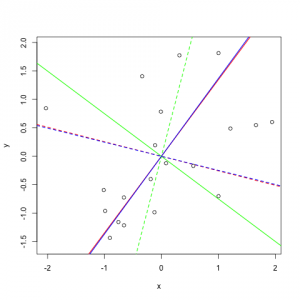This figure shows different solutions of the ICA algorithm due to some randomness. Two lines per color show the two ICs (solid and dashed) of one solution.

## 5 thoughts on “[Dimensionality Reduction #1] Understanding PCA and ICA using R”

1.akkaynt says:

Is it valid to reduce dimensionality of the data with PCA before running nonlinear dimensionality reduction?

2.Marji says:

Is PCA a good approach for reducing dimensionality in a high-dimensional multivariate time series?

1.Verena says:

Hi, I haven’t used PCA on high-dimensional multivariate time series but PLS (partial least squares) which also reduces dimensionality in a very similar way. PLS works pretty good for many applications, so I can imagine that PCA will also work but it always depends on the data! Can you provide more details?

1.Eric R Scott says:

I’m also curious as to how it compares with PLS. PLS is supervised and is prone to overfitting, so that’s one obvious difference. It’s not clear to me from this post what “independent components” are. Does that mean variables are only included in one component? (as opposed to PCA where all components include all variables, just weighted differently).

3.bryan says:

Why ICA1 & IC5 are the most important figure1 !!!!!